# Engineering Mechanics - Force System Resultants

### Exercise :: Force System Resultants - General Questions

• Force System Resultants - General Questions
26.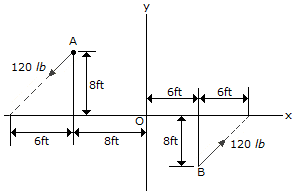Determine the magnitude and direction of the couple moment.

 A. M = 3900 lb-ft CW B. M = 3900 lb-ft CCW C. M = 3120 lb-ft CW D. M = 3120 lb-ft CCW

Explanation:

No answer description available for this question. Let us discuss.

27.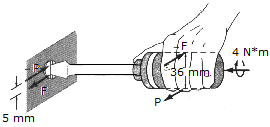A twist of 4 N-m is applied to the handle of the screwdriver. Resolve this couple moment into a pair of couple forces F exerted on the handle.

 A. P = 20 N B. P = 10 N C. P = 800 N D. P = 1600 N

Explanation:

No answer description available for this question. Let us discuss.

28.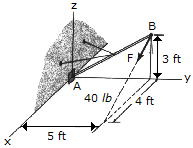Replace the force F, having a magnitude of F = 40 lb and acting at B, by an equivalent force and couple moment at A.

 A. F = (32i-24k) lb, M = (-120i+96j-160k) lb-ft B. F = (32i-24k) lb, M = 233k lb-ft C. F = (32i-24k) lb, M = -72k lb-ft D. F = (32i-24k) lb, M = (-120i+160j+40k) lb-ft

Explanation:

No answer description available for this question. Let us discuss.

29.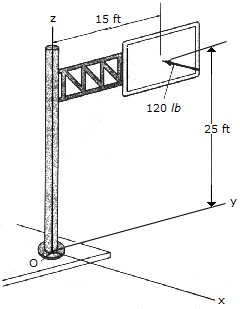The resultant force of a wind loading acts perpendicular to the face of the sign as shown. Replace this force by an equivalent force and couple moment acting at point O.

 A. F = -120i lb, M = (-3000j+1800k) lb-ft B. F = -120i lb, M = 3500i lb-ft C. F = -120i lb, M = -3500j lb-ft D. F = -120i lb, M = 3500k lb-ft

Explanation:

No answer description available for this question. Let us discuss.

30.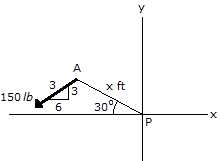Determine the magnitude and direction of the moment of the force at A about point P.

 A. Mp = 143.5 ft-lb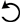B. Mp = 1191 ft-lbC. Mp = 1104 ft-lbD. Mp = 1200 ft-lb